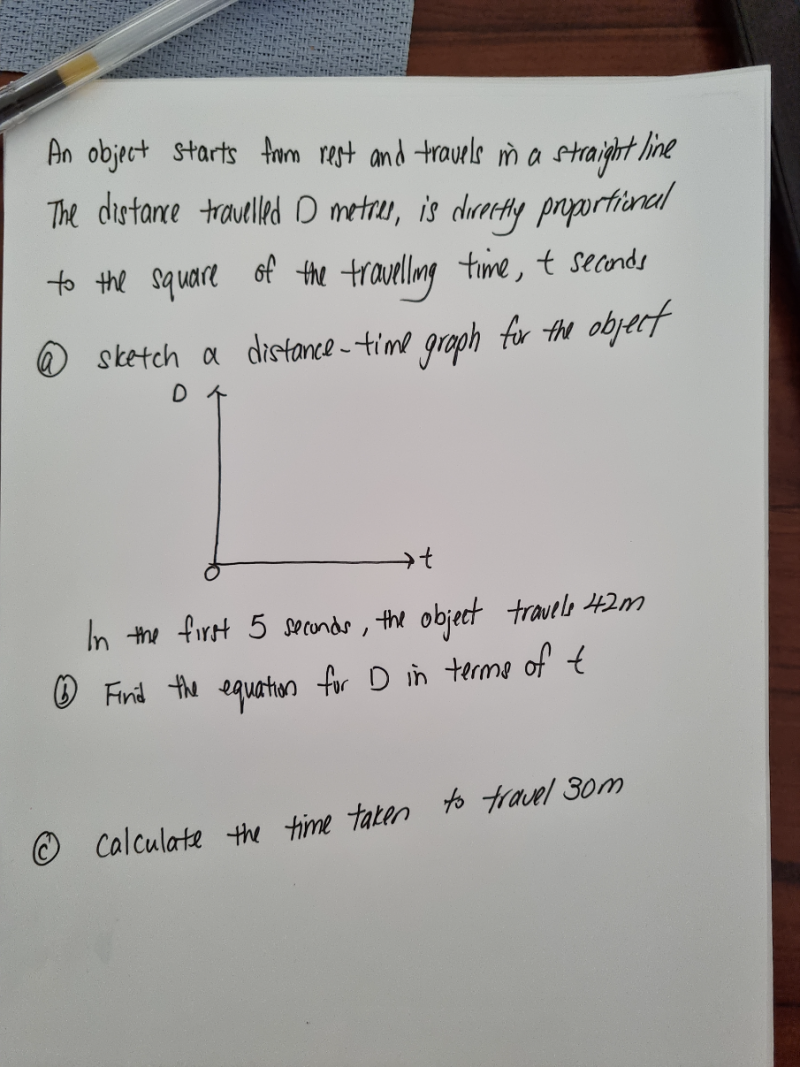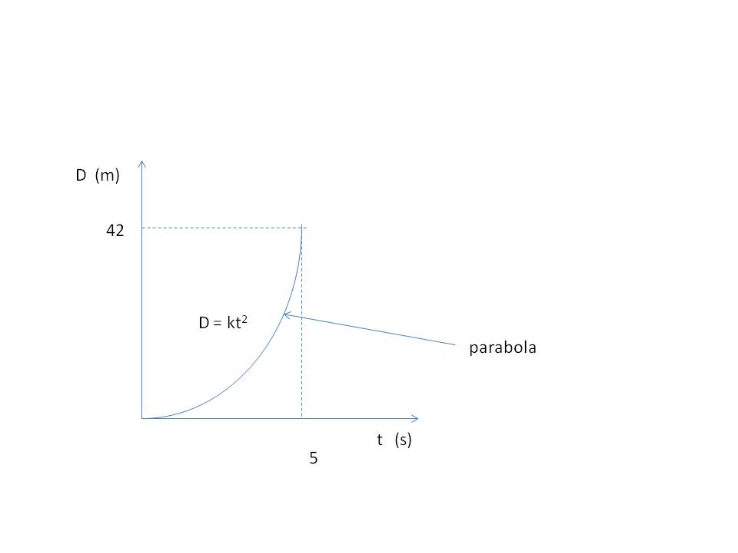# QuestionThank you.(b)  When t = 5, D = 42

D = kt2  where k is a constant.

k = D/t2 = 42 / 25  = 1.68

Therefore equation is   D = 1.68t2

(c) When D = 30,

1.68t2  = 30

t2  = 30/1.68  => t = 4.23 s

0 Replies 1 Like